Moving Towards Automating Model Selection Using Bayesian Optimization

This example shows how to build multiple classification models for a given training data set, optimize their hyperparameters using Bayesian optimization, and select the model that performs the best on a test data set.

Training several models and tuning their hyperparameters can often take days or weeks. Creating a script to develop and compare multiple models automatically can be much faster. You can also use Bayesian optimization to speed up the process. Instead of training each model with different sets of hyperparameters, you select a few different models and tune their default hyperparameters using Bayesian optimization. Bayesian optimization finds an optimal set of hyperparameters for a given model by minimizing the objective function of the model. This optimization algorithm strategically selects new hyperparameters in each iteration and typically arrives at the optimal set of hyperparameters more quickly than a simple grid search. You can use the script in this example to train several classification models using Bayesian optimization for a given training data set and identify the model that performs best on a test data set.

Alternatively, to choose a classification model automatically across a selection of classifier types and hyperparameter values, use fitcauto. For an example, see Automated Classifier Selection with Bayesian and ASHA Optimization.

This example uses the 1994 census data stored in census1994.mat. The data set consists of demographic data from the US Census Bureau to predict whether an individual makes over \$50,000 per year. The classification task is to fit a model that predicts the salary category of people given their age, working class, education level, marital status, race, and so on.

Load the sample data census1994 and display the variables in the data set.

whos
Name                 Size              Bytes  Class    Attributes

Description         20x74               2960  char

census1994 contains the training data set adultdata and the test data set adulttest. For this example, to reduce the running time, subsample 5000 training and test observations each, from the original tables adultdata and adulttest, by using the datasample function. (You can skip this step if you want to use the complete data sets.)

NumSamples = 5000;
s = RandStream('mlfg6331_64'); % For reproducibility

Preview the first few rows of the training data set.

ans=8×15 table
age     workClass       fnlwgt       education      education_num      marital_status         occupation         relationship     race      sex      capital_gain    capital_loss    hours_per_week    native_country    salary
___    ___________    __________    ____________    _____________    __________________    _________________    ______________    _____    ______    ____________    ____________    ______________    ______________    ______

39     Private          4.91e+05    Bachelors            13          Never-married         Exec-managerial      Other-relative    Black    Male           0               0                45          United-States     <=50K
25     Private        2.2022e+05    11th                  7          Never-married         Handlers-cleaners    Own-child         White    Male           0               0                45          United-States     <=50K
24     Private        2.2761e+05    10th                  6          Divorced              Handlers-cleaners    Unmarried         White    Female         0               0                58          United-States     <=50K
51     Private        1.7329e+05    HS-grad               9          Divorced              Other-service        Not-in-family     White    Female         0               0                40          United-States     <=50K
54     Private        2.8029e+05    Some-college         10          Married-civ-spouse    Sales                Husband           White    Male           0               0                32          United-States     <=50K
53     Federal-gov         39643    HS-grad               9          Widowed               Exec-managerial      Not-in-family     White    Female         0               0                58          United-States     <=50K
52     Private             81859    HS-grad               9          Married-civ-spouse    Machine-op-inspct    Husband           White    Male           0               0                48          United-States     >50K
37     Private        1.2429e+05    Some-college         10          Married-civ-spouse    Adm-clerical         Husband           White    Male           0               0                50          United-States     <=50K

Each row represents the attributes of one adult, such as age, education, and occupation. The last column salary shows whether a person has a salary less than or equal to \$50,000 per year or greater than \$50,000 per year.

Understand Data and Choose Classification Models

Statistics and Machine Learning Toolbox™ provides several options for classification, including classification trees, discriminant analysis, naive Bayes, nearest neighbors, support vector machines (SVMs), and classification ensembles. For the complete list of algorithms, see Classification.

Before choosing the algorithms to use for your problem, inspect your data set. The census data has several noteworthy characteristics:

• The data is tabular and contains both numeric and categorical variables.

• The data contains missing values.

• The response variable (salary) has two classes (binary classification).

Without making any assumptions or using prior knowledge of algorithms that you expect to work well on your data, you simply train all the algorithms that support tabular data and binary classification. Error-correcting output codes (ECOC) models are used for data with more than two classes. Discriminant analysis and nearest neighbor algorithms do not analyze data that contains both numeric and categorical variables. Therefore, the algorithms appropriate for this example are SVMs, a decision tree, an ensemble of decision trees, and a naive Bayes model.

Build Models and Tune Hyperparameters

To speed up the process, customize the hyperparameter optimization options. Specify 'ShowPlots' as false and 'Verbose' as 0 to disable plot and message displays, respectively. Also, specify 'UseParallel' as true to run Bayesian optimization in parallel, which requires Parallel Computing Toolbox™. Due to the nonreproducibility of parallel timing, parallel Bayesian optimization does not necessarily yield reproducible results.

hypopts = struct('ShowPlots',false,'Verbose',0,'UseParallel',true);

Start a parallel pool.

poolobj = gcp;

You can fit the training data set and tune parameters easily by calling each fitting function and setting its 'OptimizeHyperparameters' name-value pair argument to 'auto'. Create the classification models.

% SVMs: SVM with polynomial kernel & SVM with Gaussian kernel
'OptimizeHyperparameters','auto','HyperparameterOptimizationOptions', hypopts);
'OptimizeHyperparameters','auto','HyperparameterOptimizationOptions', hypopts);

% Decision tree
'OptimizeHyperparameters','auto','HyperparameterOptimizationOptions', hypopts);

% Ensemble of Decision trees
'OptimizeHyperparameters','auto','HyperparameterOptimizationOptions', hypopts);

% Naive Bayes
'OptimizeHyperparameters','auto','HyperparameterOptimizationOptions', hypopts);
Warning: It is recommended that you first standardize all numeric predictors when optimizing the Naive Bayes 'Width' parameter. Ignore this warning if you have done that.

Plot Minimum Objective Curves

Extract the Bayesian optimization results from each model and plot the minimum observed value of the objective function for each model over every iteration of the hyperparameter optimization. The objective function value corresponds to the misclassification rate measured by five-fold cross-validation using the training data set. The plot compares the performance of each model.

figure
hold on
N = length(mdls);
for i = 1:N
mdl = mdls{i};
results = mdls{i}.HyperparameterOptimizationResults;
plot(results.ObjectiveMinimumTrace,'Marker','o','MarkerSize',5);
end
names = {'SVM-Polynomial','SVM-Gaussian','Decision Tree','Ensemble-Trees','Naive Bayes'};
legend(names,'Location','northeast')
title('Bayesian Optimization')
xlabel('Number of Iterations')
ylabel('Minimum Objective Value')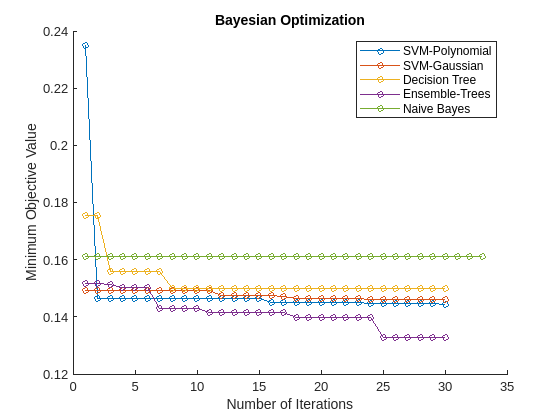Using Bayesian optimization to find better hyperparameter sets improves the performance of models over several iterations. In this case, the plot indicates that the ensemble of decision trees has the best prediction accuracy for the data. This model performs well consistently over several iterations and different sets of Bayesian optimization hyperparameters.

Check Performance with Test Set

Check the classifier performance with the test data set by using the confusion matrix and the receiver operating characteristic (ROC) curve.

Find the predicted labels and the score values of the test data set.

label = cell(N,1);
score = cell(N,1);
for i = 1:N
end

Confusion Matrix

Obtain the most likely class for each test observation by using the predict function of each model. Then compute the confusion matrix with the predicted classes and the known (true) classes of the test data set by using the confusionchart function.

figure
c = cell(N,1);
for i = 1:N
subplot(2,3,i)
title(names{i})
end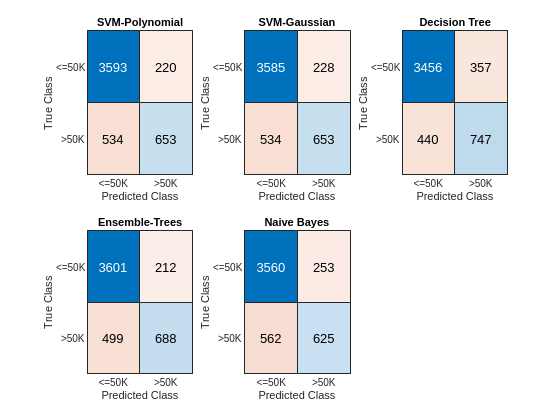The diagonal elements indicate the number of correctly classified instances of a given class. The off-diagonal elements are instances of misclassified observations.

ROC Curve

Inspect the classifier performance more closely by plotting an ROC curve for each classifier. Use the perfcurve function to obtain the X and Y coordinates of the ROC curve and the area under the curve (AUC) value for the computed X and Y.

To plot the ROC curves for the score values corresponding to the label '<=50K', check the column order of the score values returned from the predict function. The column order is the same as the category order of the response variable in the training data set. Display the category order.

c = 2×1 cell
{'<=50K'}
{'>50K' }

Plot the ROC curves.

figure
hold on
AUC = zeros(1,N);
for i = 1:N
plot(X,Y)
end
title('ROC Curves')
xlabel('False Positive Rate')
ylabel('True Positive Rate')
legend(names,'Location','southeast')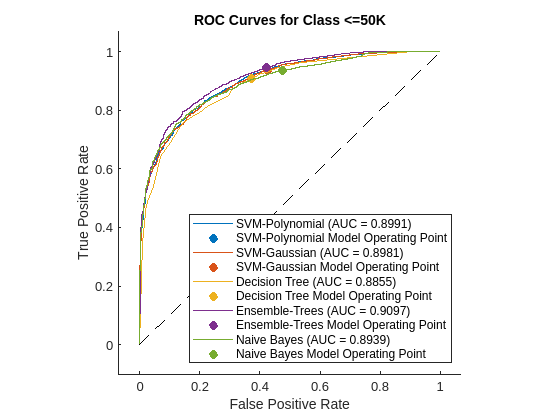An ROC curve shows the true positive rate versus the false positive rate (or, sensitivity versus 1–specificity) for different thresholds of the classifier output.

Now plot the AUC values using a bar graph. For a perfect classifier, whose true positive rate is always 1 regardless of the thresholds, AUC = 1. For a classifier that randomly assigns observations to classes, AUC = 0.5. Larger AUC values indicate better classifier performance.

figure
bar(AUC)
title('Area Under the Curve')
xlabel('Model')
ylabel('AUC')
xticklabels(names)
xtickangle(30)
ylim([0.85,0.925])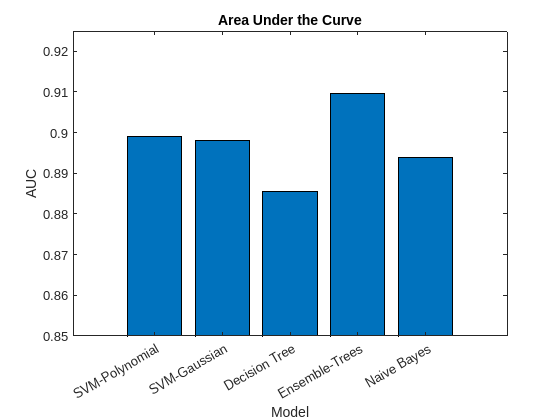Based on the confusion matrix and the AUC bar graph, the ensemble of decision trees and SVM models achieve better accuracy than the decision tree and naive Bayes models.

Resume Optimization of Most Promising Models

Running Bayesian optimization on all models for further iterations can be computationally expensive. Instead, select a subset of models that have performed well so far and continue the optimization for 30 more iterations by using the resume function. Plot the minimum observed values of the objective function for each iteration of Bayesian optimization.

figure
hold on
selectedMdls = mdls([1,2,4]);
newresults = cell(1,length(selectedMdls));
for i = 1:length(selectedMdls)
newresults{i} = resume(selectedMdls{i}.HyperparameterOptimizationResults,'MaxObjectiveEvaluations',30);
plot(newresults{i}.ObjectiveMinimumTrace,'Marker','o','MarkerSize',5)
end
title('Bayesian Optimization with resume')
xlabel('Number of Iterations')
ylabel('Minimum Objective Value')
legend({'SVM-Polynomial','SVM-Gaussian','Ensemble-Trees'},'Location','northeast')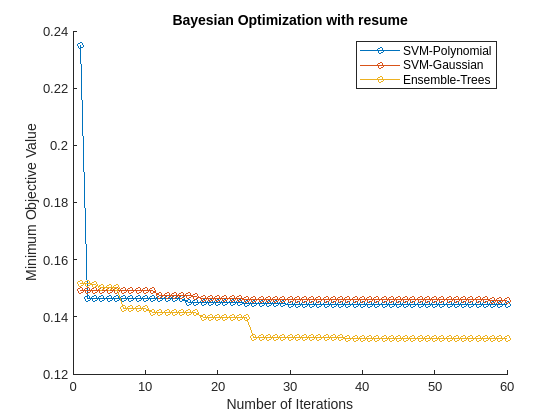The first 30 iterations correspond to the first round of Bayesian optimization. The next 30 iterations correspond to the results of the resume function. Resuming optimization is useful because the loss continues to reduce further after the first 30 iterations.